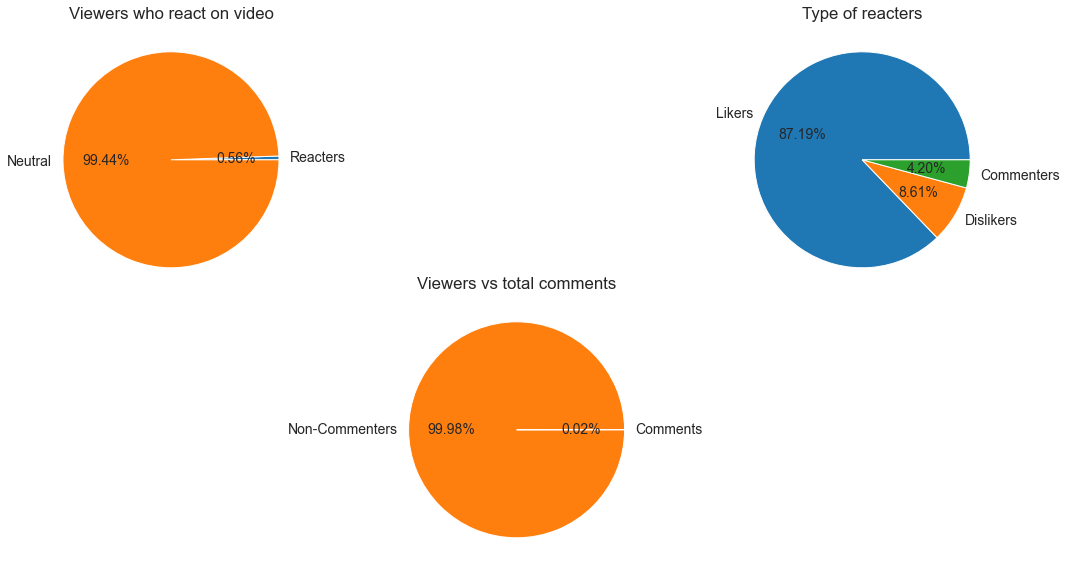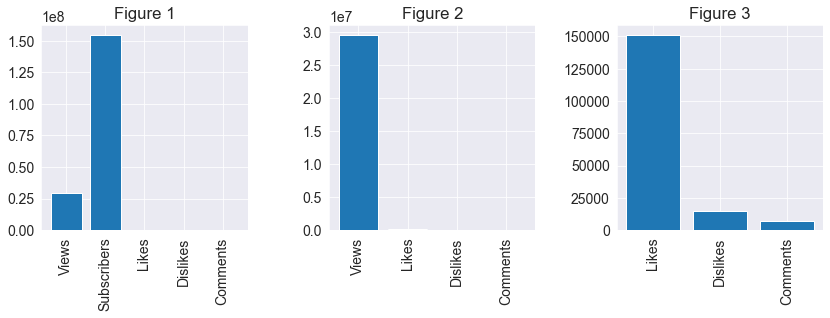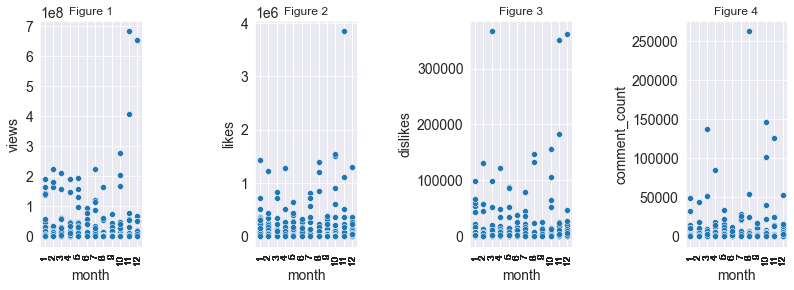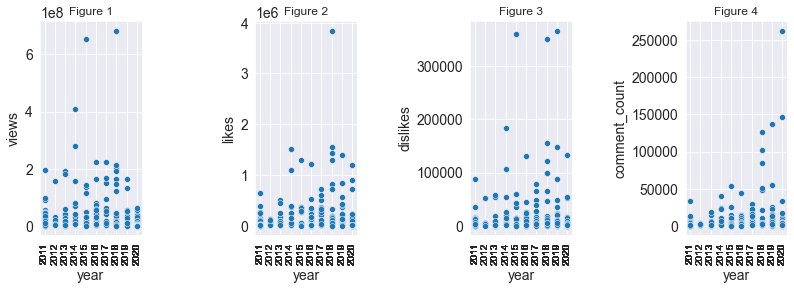Learn data science and machine learning by building real-world projects on JovianIn this project, we are going to analyse the top Youtube channel 'T-Series' using Python. First, we'll retreive videos information from this channel using Youtube Data API and Python. Then we'll create the dataset of this information using JSON and Pandas. Now, We'll analyse this dataset using Python analysis techniques and libraries ( Pandas, Matplotlib, Seaborn, etc. ) learned in Data Analysis with Python: Zero to Pandas course. I highly recommend this course if you're a beginner in this field.

#### How to run the code

This is an executable Jupyter notebook hosted on Jovian.ml, a platform for sharing data science projects. You can run and experiment with the code in a couple of ways: using free online resources (recommended) or on your own computer.

##### Option 1: Running using free online resources (1-click, recommended)

The easiest way to start executing this notebook is to click the "Run" button at the top of this page, and select "Run on Binder". This will run the notebook on mybinder.org, a free online service for running Jupyter notebooks. You can also select "Run on Colab" or "Run on Kaggle".

##### Option 2: Running on your computer locally
1. Install Conda by following these instructions. Add Conda binaries to your system `PATH`, so you can use the `conda` command on your terminal.

2. Create a Conda environment and install the required libraries by running these commands on the terminal:

``````conda create -n zerotopandas -y python=3.8
conda activate zerotopandas
pip install jovian jupyter numpy pandas matplotlib seaborn opendatasets --upgrade
``````
1. Press the "Clone" button above to copy the command for downloading the notebook, and run it on the terminal. This will create a new directory and download the notebook. The command will look something like this:
``````jovian clone notebook-owner/notebook-id
``````
1. Enter the newly created directory using `cd directory-name` and start the Jupyter notebook.
``````jupyter notebook
``````

You can now access Jupyter's web interface by clicking the link that shows up on the terminal or by visiting http://localhost:8888 on your browser. Click on the notebook file (it has a `.ipynb` extension) to open it.

We're going to gather some data from T-Series Youtube channel using Youtube Data API, JSON and Python. We'll save and export this data in csv file using Pandas.

Let's begin by importing the required libraries

In :
``````# Importing Pandas library for saving data in a dataframe and exporting it in csv file
import pandas as pd

# Importing requests, it's a Python HTTP library for making HTTP requests
import requests

# Importing JSON library to save retrieved data in json format
import json``````
##### To access Youtube Data API, we need an API key (free of cost)

In :
``````# Your API key
api_key = 'AUzaFyBzv4vr63GngXcjY-gBE4w4kjxgwwP92_w' # Replace this key with your API key``````
##### To retrieve information of T-Series Youtube channel, we require its channel ID
In :
``````# Channel ID of T-Series
channel_Id = 'UCq-Fj5jknLsUf-MWSy4_brA'``````

#### Retrieve Data

`requests.get()` is retrieving data and collecting video IDs from the url using API key and channel ID.
`json.loads()` is reading this data in text format and saving it in 'data'. We're retrieving 15 pages of videos data and each page contains maximum 50 videos information.

In :
``````# For channel's basic statistics
channel_info = requests.get(url1)
``````
##### Subscribers and available videos on this channel
In :
``````channel_subscribers = int(json_data1['items']['statistics']['subscriberCount']);
channel_videos = int(json_data1['items']['statistics']['videoCount']);

print('Total Subsribers = ',channel_subscribers,'\nTotal videos on this channel = ',channel_videos)``````
```Total Subsribers = 155000000 Total videos on this channel = 14713 ```

Now we're extracting videos and their information available on this channel. Due to API usages limitation for free google account, we're loading only 15 pages of information where each page can have maximum 50 videos information. But after increasing the API usage limit, we can just set the page limit in below code to get all the videos we want. For now, we'll analyse the channel based on this downloaded dataset only.

In :
``````limit = 15 # how many pages of information you want
video_Ids = []
nextPageToken ="" # used here to get page with unrepeated content, for 0th iteration let it be null
for i in range(limit):
for item in data['items']:
video_Id = item['id']['videoId']
video_Ids.append(video_Id)  # Storing video Ids for extracting videos information
nextPageToken = data['nextPageToken'] # to collect videos from the next page``````

Our dataset will have these columns 1. video_id 2. channel_id 3. published_date 4. video_title 5. video_description 6. likes 7. dislikes 8. views 9. comment_count

We'll save retrieved data in the categories as mentioned above, in 'data_df' dataframe

##### Note: we need to extract the data legally
In :
``````data_df = pd.DataFrame(columns=['video_id','channel_id','published_date',
'video_title','video_description',
'likes','dislikes','views','comment_count'])
``````
Out:

Let's put gathered data videos in their respective categories columns

In :
``````for i,video_Id in enumerate(video_Ids):
channel_id = data['items']['snippet']['channelId']
published_date = data['items']['snippet']['publishedAt']
video_title =  data['items']['snippet']['title']
video_description = data['items']['snippet']['description']
likes =  data["items"]["statistics"]["likeCount"]
dislikes = data["items"]["statistics"]["dislikeCount"]
views = data["items"]["statistics"]["viewCount"]
comment_count = data["items"]["statistics"]['commentCount']
row = [video_Id,channel_id,published_date,
video_title,video_description,
likes,dislikes,views,comment_count]
data_df.loc[i]=row
``````

let's save the collected data in csv format using this -

`data_df.to_csv('tseries.csv',index=False)`

By running the above code, latest dataset will be downloaded and saved in 'tseries.csv' file. To analyse the dataset and talk about its interesting points, I'm using an already downloaded dataset, so when you're checking this, you may find the information old or not up to date.

In :
``````# Importing operating system library to views files and interacting with system
import os
os.listdir() # Shows all the files available in current directory``````
Out:
``````['.DS_Store',
'tseries.csv',
'.jovianrc',
'.ipynb_checkpoints',
'zerotopandas-course-project.ipynb']``````

### Data Preparation and Cleaning

We have our raw dataset. Now, we'll remove the unwanted data, will make the dates readable and will extract the information from it (date, time, day, month year) and will store them in separate columns.

In :
``````# Storing information from csv file to Pandas dataframe
In :
``tseries_raw_df``
Out:
In :
``````# Removing unwanted columns - channel id and video id
tseries_df=tseries_raw_df.drop(['channel_id','video_id'], inplace=False,axis=1)``````
##### Raw Dataset
In :
``````# Our new dataframe with required information
tseries_df
``````
Out:
##### Making published date and time more readable
In :
``````# Importing datetime library which provides great functions to handle date and time information
import datetime
i=0
for i in range(tseries_raw_df.shape):
date_time_obj = datetime.datetime.strptime(tseries_df['published_date'].at[i], '%Y-%m-%dT%H:%M:%SZ')
tseries_df['published_date'].at[i] = date_time_obj
i = i+1``````
In :
``tseries_df``
Out:
##### Separating day, month, year, date and time from the published_date column
In :
``````i=0
date=[]
time=[]
year=[]
month=[]
day=[]
for i in range(tseries_df.shape):
d = tseries_df['published_date'][i].date();
t = tseries_df['published_date'][i].time();
y = tseries_df['published_date'][i].date().year;
m = tseries_df['published_date'][i].date().month;
da = tseries_df['published_date'][i].date().day;
date.append(d) # Storing dates
time.append(t) # Storing time
year.append(y) # Storing years
month.append(m) # Storing months
day.append(da) # Storing days
i = i+1
tseries_df.drop(['published_date'], inplace=True,axis=1)
tseries_df['published_date']=date
tseries_df['published_time']=time
tseries_df['year']=year
tseries_df['month'] = month
tseries_df['day'] = day``````
##### Cleaned dataset
In :
``````# this is our cleaned dataset, we'll use this for data analysis
tseries_df
``````
Out:
##### Size of dataset
In :
``print('Number of rows = ',tseries_df.shape,'\nNumber of columns = ',tseries_df.shape,'\nSize of the dataset = ',tseries_df.size,' elements.')``
```Number of rows = 324 Number of columns = 11 Size of the dataset = 3564 elements. ```

### Exploratory Analysis and Visualization

In this section, we'll calculate interesting parameters like sum, mean, standard deviation, range of values etc and then will see different relationships among channel statistics parameters ( views, likes, comments, dislikes etc).

##### Count, Mean, Min. value, Max. value, Standard Deviation etc.
In :
``tseries_df.describe()``
Out:
##### Total views, likes, dislikes and comments of all videos
In :
``tseries_df[['views','likes','dislikes','comment_count']].sum()``
Out:
``````views            9601791136
likes              49072840
dislikes            4844619
comment_count       2364691
dtype: int64``````
##### Average no. of views, likes, dislikes, comments on each video
In :
``````AvgLikes = tseries_df.describe()['likes']['mean']
AvgDislikes = tseries_df.describe()['dislikes']['mean']
AvgViews = tseries_df.describe()['views']['mean']
print('Average number of views on video = ',AvgViews,'\nAverage number of likes on video = ',AvgLikes,'\nAverage number of dislikes on video = ',AvgDislikes,'\nAverage number of comments on video = ',AvgComments,'\n')``````
```Average number of views on video = 29635157.827160493 Average number of likes on video = 151459.38271604938 Average number of dislikes on video = 14952.527777777777 Average number of comments on video = 7298.429012345679 ```
##### Importing plotting libraries
In :
``````# Importing Seaborn library to visualize attractive and informative statistical graphs, it's based on Matplotlib library
import seaborn as sns

# Importing Matplotlib library which is used for static, interactive, animated plotting
import matplotlib

# Importing Pyplot library which is used for 2D plotting
import matplotlib.pyplot as plt
%matplotlib inline

sns.set_style('darkgrid')
matplotlib.rcParams['font.size'] = 14
matplotlib.rcParams['figure.figsize'] = (12, 5)
matplotlib.rcParams['figure.facecolor'] = '#00000000'``````

#### Relationship among statistics parameters using Pie Charts

In :
``````fig = plt.figure()

ax1 = fig.add_axes([0, 0, 0.75, 0.75], aspect=1) # add_axes([left, bottom, width, height],aspect=1)
# Viewers who react on videos
pie_vars = ['Reacters','Neutral'];
pie_values = [tseries_df['likes'].sum()+tseries_df['dislikes'].sum(),tseries_df['views'].sum()-(tseries_df['likes'].sum()+tseries_df['dislikes'].sum())]
ax1.pie(pie_values,labels=pie_vars,autopct='%1.2f%%');
ax1.set_title('Viewers who react on video')

ax2 = fig.add_axes([0.8, 0, 0.75, 0.75], aspect=1)
# Pie chart of reacters
pie_vars = ['Likers','Dislikers','Commenters'];
pie_values = [tseries_df['likes'].sum(),tseries_df['dislikes'].sum(),tseries_df['comment_count'].sum()]
ax2.pie(pie_values,labels=pie_vars,autopct='%1.2f%%');
ax2.set_title('Type of reacters')

ax3= fig.add_axes([0.4, -0.75, 0.75, 0.75], aspect=1)
# Pie chart of commenters vs non commenters with respect to total viewers
pie_values = [tseries_df['comment_count'].sum(),tseries_df['views'].sum()-tseries_df['comment_count'].sum()]
ax3.pie(pie_values,labels=pie_vars,autopct='%1.2f%%');

plt.show()
``````Insights:
1. We can see that 99.44% of the people don't even react on T-Series videos. Only a tiny percentage of people like, dislike or comment on this channel's videos.
2. 87.19% people likes videos on this channel according to the reacters.
3. 8.61% people don't like videos on this channel.
4. People who comments on T-Series videos are less than 4.20% as someone can comment multiple times.

#### Relationship among statistics parameters using Histograms

In :
``````# Histogram of number of subscribers vs avg. viewers vs avg. likers vs avg. dislikers vs avg. commenters
fig, (ax1, ax2, ax3) = plt.subplots(1, 3)
bar_values = [tseries_df.describe()['views']['mean'],channel_subscribers,tseries_df.describe()['likes']['mean'],tseries_df.describe()['dislikes']['mean'],tseries_df.describe()['comment_count']['mean']]
ax1.bar(bar_vars,bar_values);
ax1.set_xticks(bar_vars)
ax1.set_xticklabels(bar_vars,rotation=90)
ax1.set_title('Figure 1')

bar_values = [tseries_df.describe()['views']['mean'],tseries_df.describe()['likes']['mean'],tseries_df.describe()['dislikes']['mean'],tseries_df.describe()['comment_count']['mean']]
ax2.bar(bar_vars,bar_values);
ax2.set_xticks(bar_vars)
ax2.set_xticklabels(bar_vars,rotation=90)
ax2.set_title('Figure 2')

bar_values = [tseries_df.describe()['likes']['mean'],tseries_df.describe()['dislikes']['mean'],tseries_df.describe()['comment_count']['mean']]
ax3.bar(bar_vars,bar_values);
ax3.set_xticks(bar_vars)
ax3.set_xticklabels(bar_vars,rotation=90)
ax3.set_title('Figure 3')

``````Insights:
1. T-Series has 155 million subscribers but only around 20% subscribers watch its videos or may be less than that as some of the viewers not even subscribed the channel.
2. Average number of likes, dislikes and comments on videos are negligible with respect to the number of subsribers and Viewers(Figure 1&2).
3. We can see the ratio of average number of likes, dislikes and comments on each video of T-Series.

#### Monthwise Statistics

In :
``tseries_df.groupby('month')['month'].count()``
Out:
``````month
1     21
2     23
3     18
4     22
5     81
6     15
7     17
8     26
9     36
10    24
11    17
12    24
Name: month, dtype: int64``````

Insights:
1. T-Series uploads highest number of videos in month of 'May' which is two-three times more than videos being uploaded in other months.
2. T-Series uploads lowest number of videos in month of 'June'.

##### Monthwise total views, likes, dislikes and comments
In :
``tseries_df.groupby(tseries_df['month']).sum()``
Out:
##### Monthwise statistics using scatterplots
In :
``````# Importing sys module which provides access to some variables used or maintained by the interpreter and to functions that interact strongly with the interpreter.
import sys

if not sys.warnoptions:
# Importing warnings library to handle warnings
import warnings
warnings.simplefilter("ignore")

fig, (ax1, ax2, ax3, ax4) = plt.subplots(1, 4)

# Monthwise statistics of views
sns.scatterplot(tseries_df['month'],tseries_df['views'],ax=ax1)
ax1.set_title('Figure 1',fontsize=12)
ax1.set_xticks(tseries_df['month'])
ax1.set_xticklabels(tseries_df['month'],rotation=90,fontsize=10)

# Monthwise statistics of likes
sns.scatterplot(tseries_df['month'],tseries_df['likes'],ax=ax2)
ax2.set_title('Figure 2',fontsize=12)
ax2.set_xticks(tseries_df['month'])
ax2.set_xticklabels(tseries_df['month'],rotation=90,fontsize=10)

# Monthwise statistics of dislikes
sns.scatterplot(tseries_df['month'],tseries_df['dislikes'],ax=ax3)
ax3.set_title('Figure 3',fontsize=12)
ax3.set_xticks(tseries_df['month'])
ax3.set_xticklabels(tseries_df['month'],rotation=90,fontsize=10)

sns.scatterplot(tseries_df['month'],tseries_df['comment_count'],ax=ax4)
ax4.set_title('Figure 4',fontsize=12)
ax4.set_xticks(tseries_df['month'])
ax4.set_xticklabels(tseries_df['month'],rotation=90,fontsize=10)Insights:
1. T-Series uploaded its most viewed video in month of 'November'.
2. T-Series uploaded its most liked video in month of 'November'.
3. T-Series uploaded its most disliked video in month of 'March'.
4. T-Series uploaded its most commented video in month of 'August'.

#### Yearwise Statistics

In :
``tseries_df.groupby('year')['year'].count()``
Out:
``````year
2011    76
2012     7
2013    15
2014    12
2015    16
2016    31
2017    28
2018    36
2019    45
2020    58
Name: year, dtype: int64``````

Insights: T-Series uploaded highest number of videos in year 2011 and lowest number of videos in year 2012.

##### Yearwise total views, likes, dislikes and comments
In :
``tseries_df.groupby(tseries_df['year']).sum()``
Out:
##### Yearwise statistics using scatterplots
In :
``````import sys

if not sys.warnoptions:
import warnings
warnings.simplefilter("ignore")

fig, (ax1, ax2, ax3, ax4) = plt.subplots(1, 4)

# Yearwise statistics of views
sns.scatterplot(tseries_df['year'],tseries_df['views'],ax=ax1)
ax1.set_title('Figure 1',fontsize=12)
ax1.set_xticks(tseries_df['year'])
ax1.set_xticklabels(tseries_df['year'],rotation=90,fontsize=10)

# Yearwise statistics of likes
sns.scatterplot(tseries_df['year'],tseries_df['likes'],ax=ax2)
ax2.set_title('Figure 2',fontsize=12)
ax2.set_xticks(tseries_df['year'])
ax2.set_xticklabels(tseries_df['year'],rotation=90,fontsize=10)

# Yearwise statistics of dislikes
sns.scatterplot(tseries_df['year'],tseries_df['dislikes'],ax=ax3)
ax3.set_title('Figure 3',fontsize=12)
ax3.set_xticks(tseries_df['year'])
ax3.set_xticklabels(tseries_df['year'],rotation=90,fontsize=10)

sns.scatterplot(tseries_df['year'],tseries_df['comment_count'],ax=ax4)
ax4.set_title('Figure 4',fontsize=12)
ax4.set_xticks(tseries_df['year'])
ax4.set_xticklabels(tseries_df['year'],rotation=90,fontsize=10)Insights:
1. T-Series uploaded its most viewed video in the year '2018'.
2. T-Series uploaded its most liked video in the year '2018'.
3. T-Series uploaded its most disliked video in the year '2019'.
4. T-Series uploaded its most commented video in in the year '2020'.

##### Top 10 most viewed videos from the dataset
In :
``tseries_df.sort_values(by='views',ascending=False).head(10)``
Out:
##### Top 10 least viewed videos
In :
``tseries_df.sort_values(by='views',ascending=True).head(10)``
Out:

We've seen many relationships above. Now, we'll see some interesting questions from the above insights of plots and about the channel according to our dataset.

##### Q1. Has this Corona pandemic affected this channel till now?

According to the yearwise statistics, T-Series has uploaded 58 videos till now in year 2020 which is higher than the total number of videos uploaded in year 2019. Also, channel is doing good in terms of views, likes and comments in this year so they are able to manage the channel in this pandemic with their music content. Although they are not able to create much new video content because of this situation.

##### Q2: Most famous video of T-Series was uploaded in November, 2018. What are its title and description?
In :
``````pd.options.display.max_colwidth = 50
Out:
In :
``````pd.options.display.max_colwidth = 100
```38 Leja Re | Dhvani Bhanushali | Tanishk Bagchi | Rashmi Virag |Radhika Rao| Vinay Sapru | Siddharth Name: video_title, dtype: object ```

This is the title of the most viewed and liked song.

In :
``````pd.options.display.max_colwidth = 600 # increase this value to view full description
```38 T-Series Presents latest Hindi Video Song of 2018 "Leja Re" , sung by "Dhvani Bhanushali ",music is recreated by "Tanishk Bagchi" and the lyrics of this new song are penned by " Rashmi Virag". The video features Dhvani Bhanushali, Siddharth, Deepali Negi and Palak Singhal. The Video By Radhika Rao & Vinay Sapru. Enjoy and stay connected with us !! \n\nSUBSCRIBE 👉 http://bit.ly/TSeriesYouTube for Latest Hindi Songs 2018! \n#LejaRe #weddingsong #IndianWeddingSong \n\n♪ Available on ♪\niTunes : http://bit.ly/Leja-Re-Dhvani-Bhanushali-iTunes\nHungama : http://bit.ly/Leja-Re-Dhvani-Bhanushali... Name: video_description, dtype: object ```

This is the video description of the most viewed and liked song.

##### Q3: Which are the recent videos uploaded on this channel?
In :
``````# Latest 10 videos from the dataset
pd.options.display.max_colwidth = 50
Out:
##### Q4: Which are the oldest videos available of this channel?
In :
``tseries_df.sort_values(by='published_date',ascending=True).head(10)``
Out:
##### Q5: Which is the most commented video of this channel?
In :
``````pd.options.display.max_colwidth = 100
Out:
##### Q6: Which is the most disliked video of this channel?
In :
``````pd.options.display.max_colwidth = 100
Out:
In :
``pd.options.display.max_colwidth = 50``

### Inferences and Conclusion

In this project, we extracted Youtube channel T-Series' videos information using Youtube API, Python, JSON and requests libraries. We prepared our csv dataset using it. We cleaned this raw dataset, performed some operations to make it more convenient to use and analyse. Then we analysed different relationships among time, subscribers, views, likes, comments, dislikes etc. We asked and answered some questions based on this information.

### References and Future Work

Although we used some data only for this project but one can download the any channel's whole data by using his API appropriately and then use it for complete analysis purpose.

References:-
1. GeeksForGeeks
2. Pandas Documentation
3. stackoverflow
4. Matplotlib Documentation

In :
``import jovian``
In :
``project_name = "youtube-channel-tseries-analysis"``
In :
``jovian.commit(project=project_name, environment=None)``
```[jovian] Attempting to save notebook.. [jovian] Updating notebook "rkkasotiya/youtube-channel-tseries-analysis" on https://jovian.ml/ [jovian] Uploading notebook.. [jovian] Committed successfully! https://jovian.ml/rkkasotiya/youtube-channel-tseries-analysis ```
Out:
``'https://jovian.ml/rkkasotiya/youtube-channel-tseries-analysis'``
In [ ]:
``jovian.commit(outputs=['tseries.csv'])``
```[jovian] Attempting to save notebook.. ```
In [ ]:
`` ``